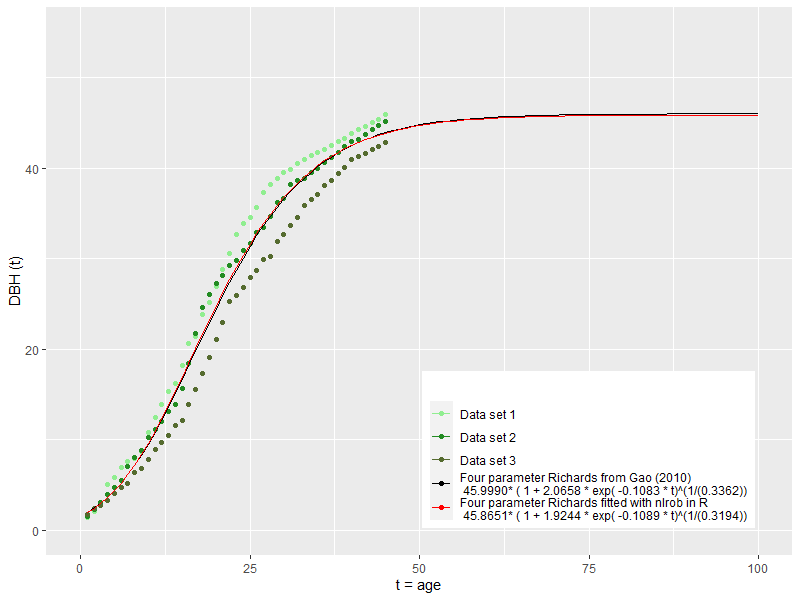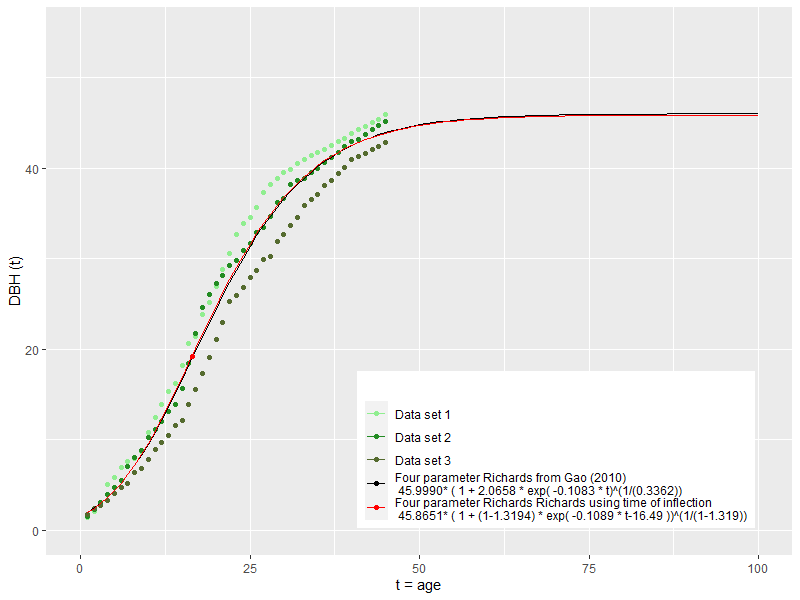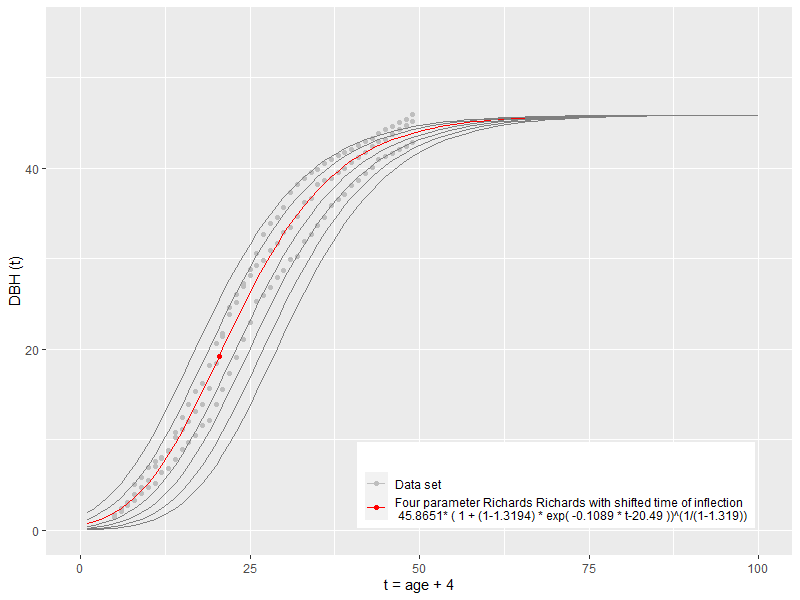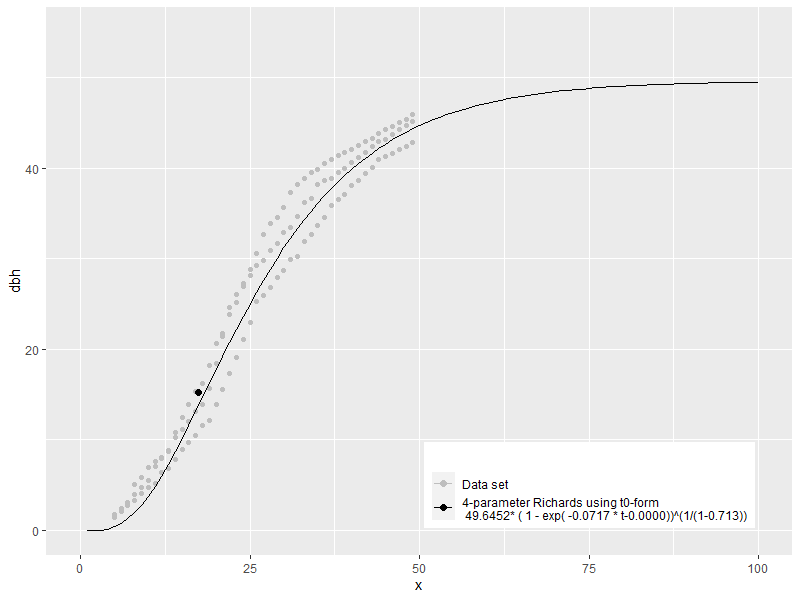# Reparametrisation of Richard growth equation for Sophora japonica

## Introduction

The Richards growth model (Richards, 1959) describes sigmoid shape curves with an upper asymptote. The model can describe many growth variables of trees (e.g., height, diameter at breast height, basal area, and volume) with initial slow growth, increasing in speed with maxima at an inflection point, and slowly approaching adult and final values. This empirical model is valued for accuracy and flexibility but at the expense of interpreting the parameters as biological values. These models have been applied in various fields in many different notations and parameterizations (Tjørve and Tjørve 2010), making it difficult to compare the results of already published studies. Reparametrization is often introduced to have specific curve characteristics as a single value (i.e., starting value, time of inflection), avoiding gaps in parameter space, and stabilizing parameter estimation by reducing intrinsic non-linearity, a problem described by Ratkowsky (1993) for this equation. The data set and estimated equation is from Gao (2010), analyzing tree rings from core samples from S.j at the campus of the Agriculture University in Anhui, Hefei, China.

## Dataset and original equation

Gao (2010) gave the equation in the form:

DBH(t) = 45.9999 /( ( 1 + 2.0658* exp( -0.108270* t) )^( 1 /0.336217))

 DBH(t) = A/(( 1+S*exp(-k* t))^(1/m)); sigmoid m > -1

with:
A = 45.999
S = 2.0658
k = 0.108270
m = 0.336217

with interception of y-axis at:
DBH(T0) = 1.64

and inflection point:
Ti  = 16.78; DBH(Ti) = 19.42


This model was refitted in R with robust non-linear regression for testing, leading to almost identical parameters (Figure 1).The result shows at least one unrealistic parameter. The curve has a value of 1.64 at T0, but DBH, by definition, can not be larger than zero at time zero. The first measurable annual ring at DBH for Sophora japonica may only occur around four to six years after germination. It is evident that the data set is biased by associating the first measurable DBH from a core with the age of 1 year. Age for DBH extracted from cores need to be corrected to estimate the germination and lag time between germination and the occurrence of values for DBH. Based on observation of trees in tree nurseries, the first measurable DBH from coring (ca. 1.5 cm) is most likely in age of five. The increment of 4 years shifts the data points to the right (x1 = 5 and the growth model need to be shifted accordingly.

## Reparametrization to Ti form

In the equation used, S is the single parameter that moves the curve horizontally without changing the curves other characteristics, but the parameter is hard to interpret. Therefore a reparameterized form is used, where the inflection time becomes the single parameter that moves the curve horizontally and replacing m with (1-d), to allow only positive values. This form is known as Ti form (Tjørve and Tjørve, 2010).

equation  can be also written as:

 DBH(t) = A*(( 1+S*exp( -k* t) )^( -1 /m))

with inflection point:
Ti = log (S/m)/k; DBH(Ti ) =  A / ( 1+m)^(1/m)

substituting:
S = exp (Ti * k) * m  and  m = d-1

in equation  yields:
DBH(t) = A*(( 1 + exp (Ti * k) * (d-1)) * exp(-k*t) )^(-1/(d-1)))

and can be simplified to:
 DBH(t) = A * (( 1 + (d-1) * exp(- k * (t-Ti)))^( 1 /(1-d)))The curve has the same characteristics as the original curve but allows incremental shifts along the x-axis by modifying Ti (Figure 2).Reparametrization and subsequent shifting does not change the curve's shape. Even with a further shift, there would always be small values for DBH remaining before the tree reaches breast height (1.4 m). Richard's models in the form of equation  always lead to intersections of the y-axis or start value at germination. They are also restricted to inflection points with DBH(Ti) > A/e.

## Refitting to the complementary (T0) form

As Richards models with equation  are not covering forms with a start point at the x-axis at a lag time where DBH (T0) = 0, the complementary form (Richards, 1959; Tjørve and Tjørve, 2010) with following equation os needed:

for equation  the complemantary form is:
 DBH(t) = A*((1-S*exp(-k*t) )^(1/m)); sigmoid 0 < m < 1

with:
T0 = -log(1/S)/k
and inflection point:
Ti = log(S/m)/k; DBH(Ti) =  A/(1+m)^(1/m)


Again, parameter S is hard to understand and validate directly. Therefore a reparameterized form is used, where the start time T0 becomes the single parameter that moves the curve horizontally.

substituting:
S = 1 / exp (T0 * k)
in equation  yields:
DBH(t) = A*(( 1-(1/exp (T0*k))*exp( -k* t) )^(1/m));

and can be simpliefied to:
 DBH(t) = A* ( 1-exp (-k*(t- T0)))^(1/m)); sigmoid 0 < m < 1


Fitting with robust regression leads to:The resulting curve has an almost identical form, even the parameters showing notable differences. Using this form of the model restricts values for d to 0 < d < 1 and subsequently to a value with DBH(Ti) < A/e.

## Reparametrization of the T0 form to the Ti form

The new equation is also reparameterized to a form with the inflection time Ti as a single parameter to create comparable parameters, leading to the same form as the equation .

S = exp (Ti * k) * m  and  m = d-1
in equation  yields:
DBH(t) = A*((1-(exp (Ti*k)*(d-1)))*exp( -k* t) )^(1/(d-1)));

and can be simpliefied to:
DBH(t) = A * (( 1 + (d-1) * exp(- k * (t-Ti)))^( 1 /(1-d)))


The reparametrization leads to the same eqaution as Equation  covers both complementary forms. Almost all Richards forms can be reparameterized to this form, allowing parameter comparison with other studies. The equation is sigmoid for d>0, has a starting point at the x-axis for 0 < d < 1, and has a starting point at the y-axis for d > 1. The inflection point relative value is only determined by a single parameter (d) in this form. The parameter space for d has no leap, but a point of singularity, as at d=1, the function is not defined. Fitting with to this form, covering the entire parameter space will provide the best fitting form when starting parameter selected correctly.

## Refitting with Ti-form

Fitting data directly to equaution  leads to a result identically with fitting to equation  after repararemeterization. The result can not paramerized to form  or  as the values for d and m are outside the functions parameter space.Here is an inherent problem with the empirical growth model visible. The best-fitting curve leads to unrealistic parameters. The "best" model does not yield the "correct" model; it is overfitted. As the inflection point value is close to A/e, it would be interesting to test the Gompertz model. Tjørve and Tjørve (2010) show that the Richards model converges to the Gompertz model for d -> 1 and DBH(i) -> A/e. In the next post, the focus will be on statistical properties for analyzing and comparing the curves in more detail.

Citations:

Richards, F. J. (1959). "A Flexible Growth Function for Empirical Use". Journal of Experimental Botany. 10 (2): 290–300. doi:10.1093/jxb/10.2.290.

Tjørve E, Tjørve KM. (2010). A unified approach to the Richards-model family for use in growth analyses: why we need only two model forms. J Theor Biol. 2010 Dec 7;267(3):417-25. doi: 10.1016/j.jtbi.2010.09.008. Epub 2010 Sep 8. PMID: 20831877.

Gao Qianfeng (2010). Study On Tree Breast － Height Diameter Growth Model for Sophora Japonica in Hefei city. Anhui Agricultural Science Bulletin 2010，16(15). DOI：10.3969/j.issn.1007-7731.2010.15.017